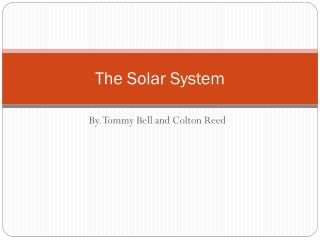DownloadDownload PresentationThe Solar System

# The Solar System

Télécharger la présentation## The Solar System

- - - - - - - - - - - - - - - - - - - - - - - - - - - E N D - - - - - - - - - - - - - - - - - - - - - - - - - - -
##### Presentation Transcript

1. The Solar System By. Tommy Bell and Colton Reed

2. Mercury • Is the closet planet to the sun • Its orbit takes 88 days and is the shortest of all the planets • It is 57900000 km from the sun • The diameter of Mercury is 4879 km • It has no satellites around it • Its surface temperature ranges from -173 to 427 Celsius • The dominant atmospheric gas is helium • The surface gravity is 0.38 • Mercury’s mass is 0.06 • Mercury is cover in craters • Mercury was once volcanic • Its atmosphere is very thin

3. Venus • The diameter is 12,104 km • Venus has no satellites • Its surface temperature is 464 Celsius • Its orbit is 225 days • Is 10820000 km from the sun • Its atmosphere is mainly made of carbon dioxide • Venus’s surface gravity is 0.91 • Its mass is .82 • Venus diameter is .95 • Venus’s distance from the sun is 0.72 Au’s • Rotates ate 35 kilometers per hour • Venus has similar size, density, and mass as earth

4. Earth • Its theThirdplanet from sun • Orbit period is 365.24 days • It completes a rotation in 23 hours and 56 minutes. • Life is possible because it is warm enough for water to exist in liquid form • Earth is 149,600,000 kilometers from the sun • 12,756 kilometers in diameter. • We have one satellite, our moon. • -13 to 37 degrees Celsius • The dominant atmospheric gases are nitrogen and oxygen. • Mass of 1.00 • Surface gravity is one • Only known planet to have life

5. Mars • It is the fourth closest planet to the sun. • Mars is 22,790,000 kilometers from the sun • Mars is 6,794 kilometers in diameter • It has an orbit period of 687 days. • Rotates once every 24 hours and 37 minutes. • Has two satellites revolving around it • Has a surface temperature of -127 to 37 degrees celsius • Carbon Dioxide is the dominant atmospheric gas. • Has a mass 0.11 • Has a surface gravity of 0.38 • Mars is made up largely of iron ore. • Orbits at a speed of 24.1

6. Jupiter • Largest planet in milky way galaxy • it is the 5th closest to the sun • First of the outer planets • Diameter of 142,984 kilometers • Orbits the sun in 11.9 earth years • Has the shortest day length of any planet in milky way: 9 hours and 50 minutes. • Has 16 satellites. • Hydrogen and Helium are the dominant atmospheric gases. • -153 degrees celsius • Jupiter is 778,300,000 kilometers away from the sun • Has a mass of 317.8 • Jupiters surface gravity is 2.53

7. Saturn • 6th closest planet to the sun • Has a diameter of 120,536 kilometers. • Saturn Orbits the sun every 29.5 years • Rotates rapidly at 10 hours 30 minutes. • 18 satellites orbit saturn. • Surface temperature of -185 degrees celsius • Hydrogen and Helium make up the atmospheres gases. • Over Half a billion kilometers away from jupiter • It has several rings surrounding it • Least dense planet in the solar system • Has a surface gravity of 1.07

8. Uranus

9. Neptune

10. Pluto